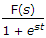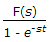# Electronics and Communication Engineering - Signals and Systems

Exercise : Signals and Systems - Section 1
6.
If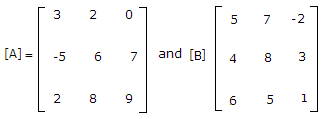and A + B is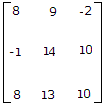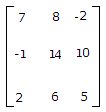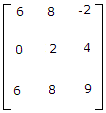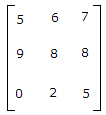Explanation:

7.
A voltage V(t) is a Gaussian ergodic random process with a mean of zero and a variance of 4 volt2. If it is measured by a dc meter. The reading will be
0
4
2
2
Explanation:

8.
A first order system will never be able to give a __________ response
1. band stop
2. band pass
3. all pass
Choose the correct option
1, 2, 3 true
1 and 3 true, 2 false
1, 2 are true 3 is false
1, 2 are false, 3 is true
Explanation:

Because to design band pass or band stop, transfer function of must be second order.

9.
In the given figure the ratio T/d is the duty factor.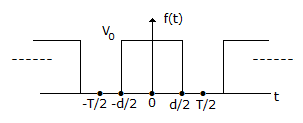True
False
Explanation: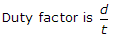.

10.
If £[f(t)] = F(s), then £[f(t - T)] =
est F(s)
e-st F(s)